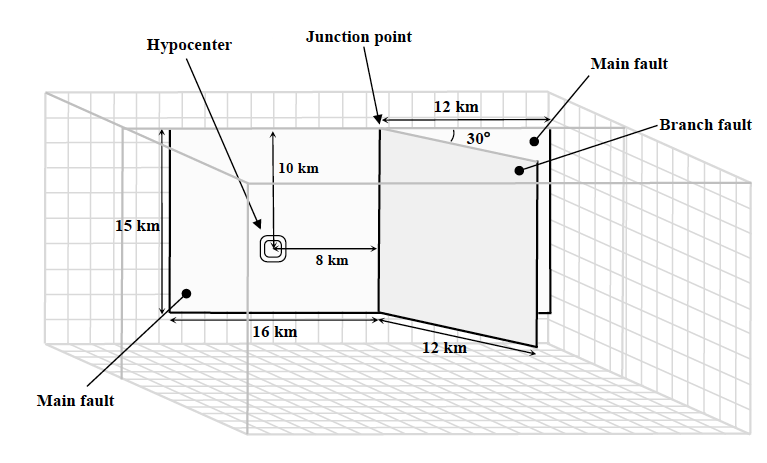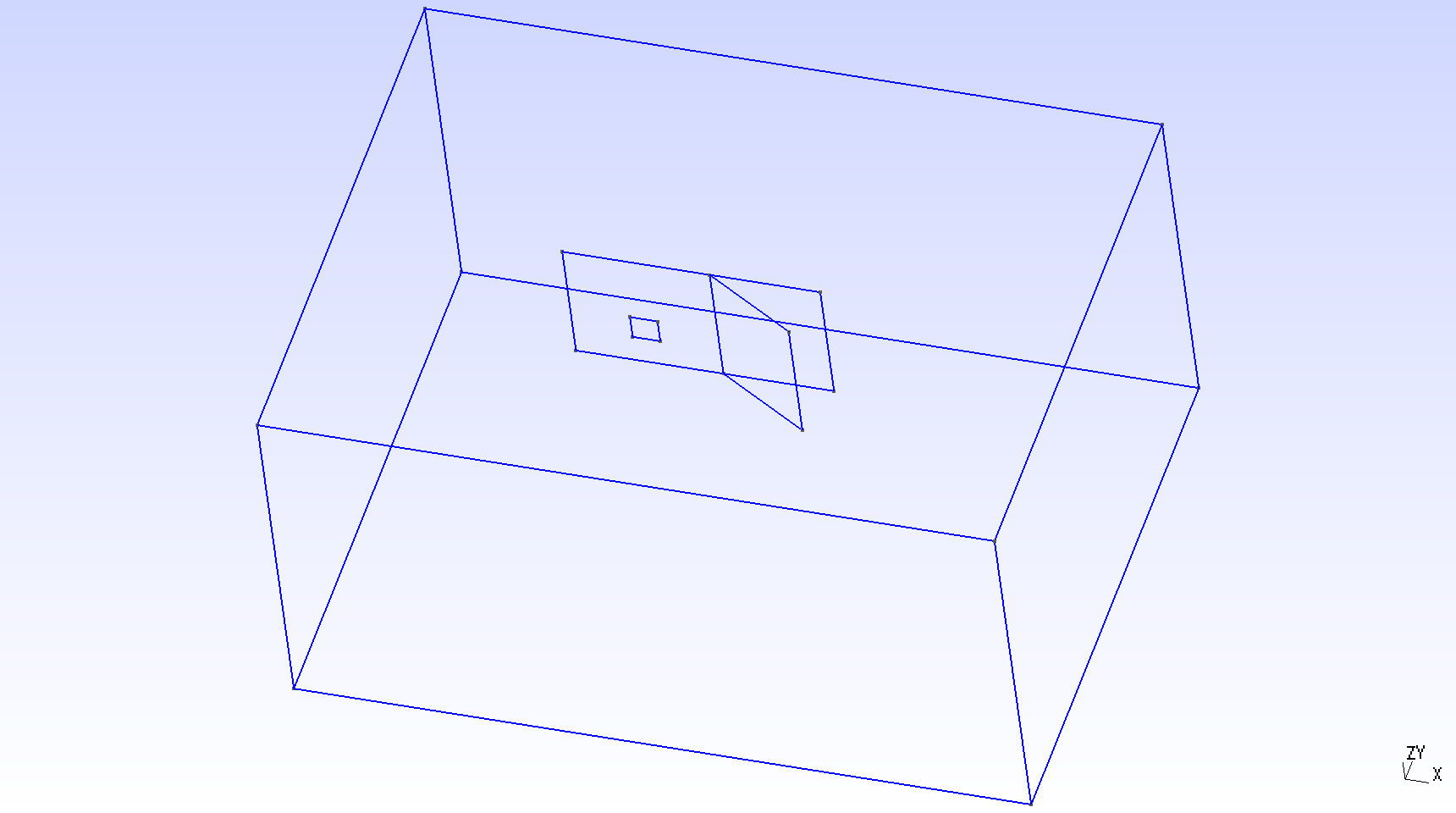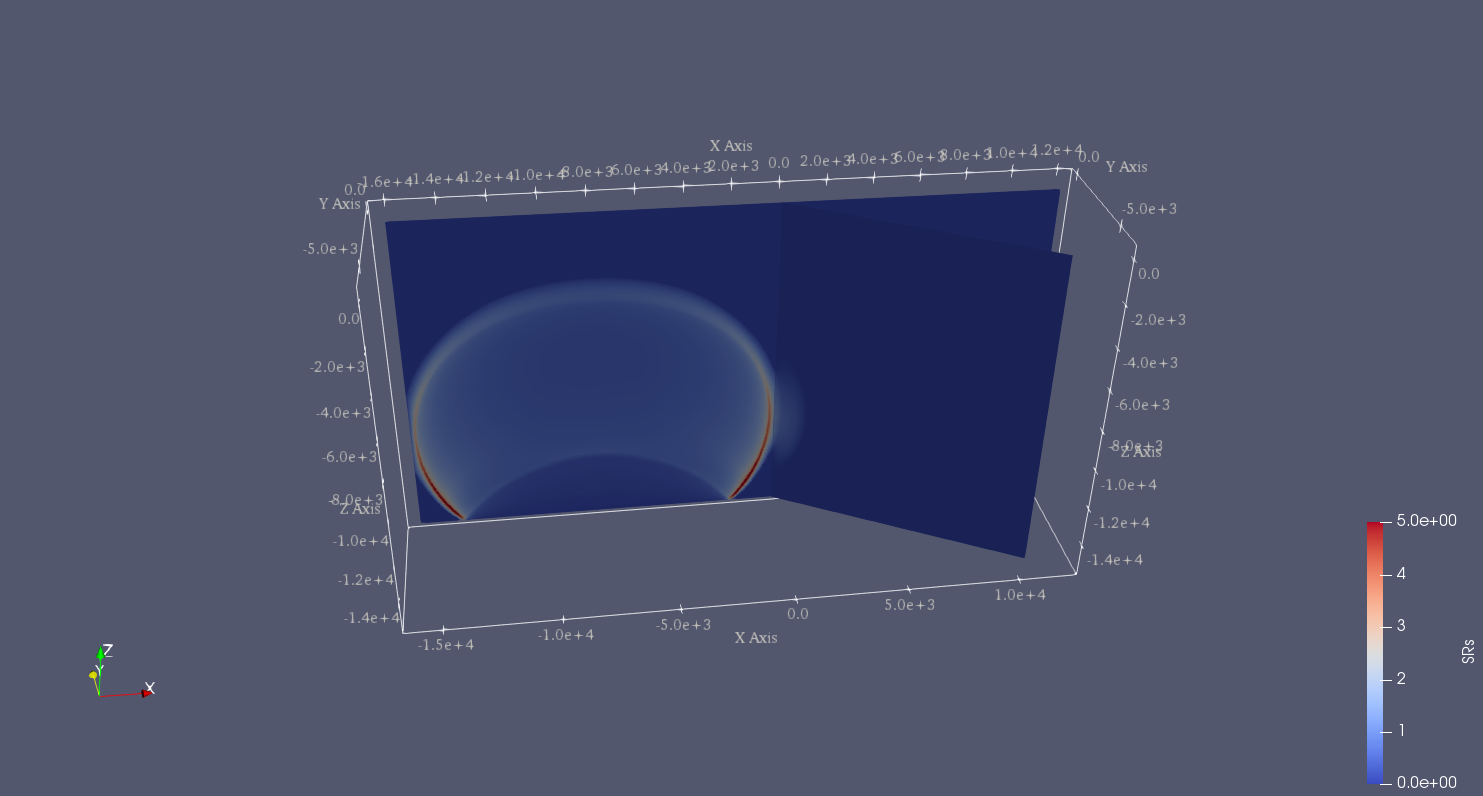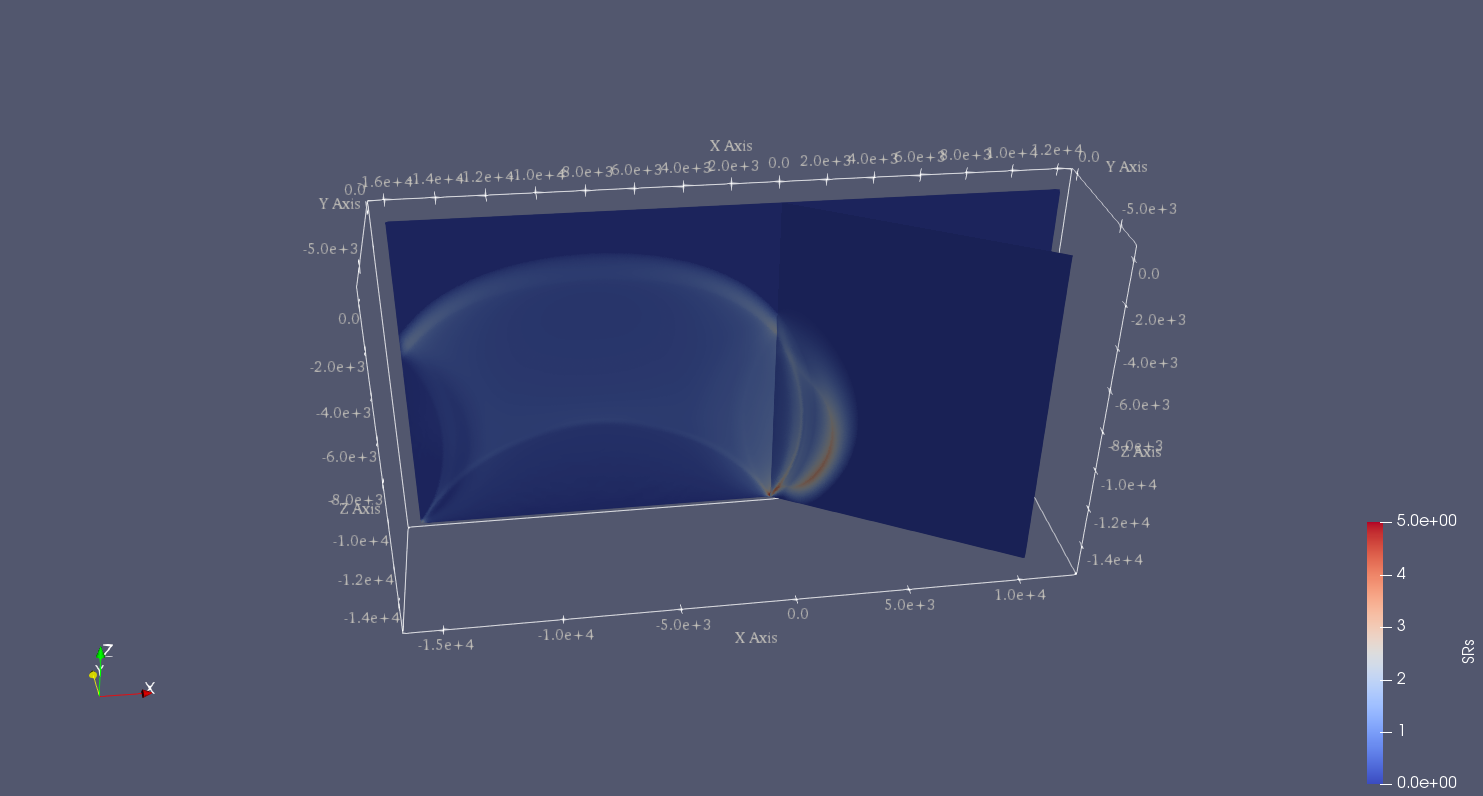# SCEC TPV24

TPV24 is designed to illustrate dynamic rupture in a fault branching system. TPV24 contains two vertical, planar strike-slip faults; the main fault and a branch fault intersecting at an angle of 30 degrees (Figure [fig:tpv24]). The earthquake rupture is artificially nucleated in a circular zone on the main fault surface and then spontaneously propagates to the branching fault.Diagram of TPV24 branching fault geometry. The main fault is (16 000 + 12 000) m and the branching fault is 12 000 m. Both faults are 15 000 m wide. The intersecting angle is 30 degrees. The nucleation patch locates at 10 000 m depth and 8000 m horizontally from the joint point.

## Geometry

There are two faults, called the main fault and the branch fault (Figure [fig:tpv24]). The two faults are vertical, planar, strike-slip faults. The faults reach the earth’s surface.

The main fault is a rectangle measuring 28 000 m along-strike and 15 000 m deep. The branch fault is a rectangle measuring 12 000 m along-strike and 15 000 m deep. There is a junction point. It is located 12 000 m from the right edge of the main fault, and the main fault passes through it.

The branch fault makes an angle of 30 degrees to the main fault. The branch fault ends at the junction point.

The hypocenter is centered along-strike at a depth of 10 km on the left side of the main fault. That is, the hypocenter is 8000 m from the junction point, and 10 000 m deep.

Figure [fig:tpv24mesh] shows the fault model generated in Gmsh. The mesh file can be generated using https://github.com/SeisSol/Examples/blob/master/tpv24/generating_the_mesh.sh.Geometry generated in Gmsh of TPV24. The main fault lies in y-plane.

## Initial stress

The initial stress condition is depth-dependent at the depth above 15600 m. Table [table:tpv24] summarizes the initial stress conditions in TPV24.

Parameter

Description

Value

Unit

s_zz

$$\sigma_{zz}$$

-2670*9.8*depth | Pa

s_xz

$$\sigma_{xz}$$

0

Pa

P_f

fluid pressure

1000*9.8*depth | Pa

s_yz

$$\sigma_{yz}$$

0.0

Pa

inside the nucleation zone

s_yy

$$b33*(\sigma_{zz} + P_f) - P_f$$

Pa

s_xx

$$b22*(\sigma_{zz} + P_f) - P_f$$

Pa

s_xy

$$b23*(\sigma_{zz} + P_f)$$

Pa

outside the nucleation zone

s_yy

$$\sigma_{zz}$$

Pa

s_xx

$$\sigma_{zz}$$

Pa

s_xy

0

Pa

Note that the effective stress tensor is :

$\begin{split}\bar{\sigma}_{effective}= \begin{bmatrix} &\sigma_{xx} + P_f , & \sigma_{xy} ,& \sigma_{xz} \\ &\sigma_{xy}, &\sigma_{yy} +P_f , &\sigma_{yz} \\ &\sigma_{xz} ,&\sigma_{yz} , &\sigma_{zz} +P_f \end{bmatrix}\end{split}$

## Nucleation parameters

Nucleation is performed by forcing the fault to rupture, within a circular zone surrounding the hypocenter. Forced rupture is achieved by artificially reducing the friction coefficient, beginning at a specified time. The parameter specifies how long it takes for the friction coefficient to be artificially reduced from its static value to its dynamic value. So, the friction coefficient reaches its dynamic value at time. We reduce the friction coefficient gradually, over an interval of time, in order to smooth the nucleation process and reduce unwanted oscillations.

$\begin{split}T = \left\{ \begin{array}{lr} & \frac{r}{0.7Vr} + \frac{0.081*r_{crit} }{0.7Vr} (\frac{1}{1-(r/r_{crit})^2} - 1), r \leq r_{crit} \\ & 1E+09, r > r_{crit}\\ \end{array} \right.\end{split}$

The cohesion zone is defined as :

$\begin{split}C_0 = \left\{ \begin{array}{lr} & 0.3 + 0.000675 * (4000 - depth), depth < 4000 m \\ & 0.3 MPa, depth \geq 4000 m\\ \end{array} \right.\end{split}$

Note that the frictional cohesion is 3.00 MPa at the earth’s surface. It is 0.30 MPa at depths greater than 4000 m, and its value is linearly tapered in the uppermost 4000 m.

The friction parameters are listed in Table [table:tpv24fric].

Parameter

Description

Value

Unit

mu_s

static friction coefficient

0.18

mu_d

dynamic friction coefficient

0.12

d_c

critical distance

0.30

m

C_0

fault cohesion

Pa

T

forced rupture time

s

t_0

forced rupture delay time

0.5

s

Table: Table of LSR parameters on the fault in TPV24.

## Results

The model is run for 12.0 seconds after nucleation. The earthquake rupture is artificially nucleated in a circular zone on the main fault surface. The rupture then spontaneously propagates on the main fault and encounters a branching fault. The branching fault continues to rupture as well as the rest main fault. The fault slip rate is shown in Figure [fig:tpv24result1].Snapshot of slip rate in branching fault system. Slip rate at 2 s.Snapshot of slip rate in branching fault system. Slip rate at 3.5 s.